# 3 D Computer Vision 3 D Vision and

• Slides: 29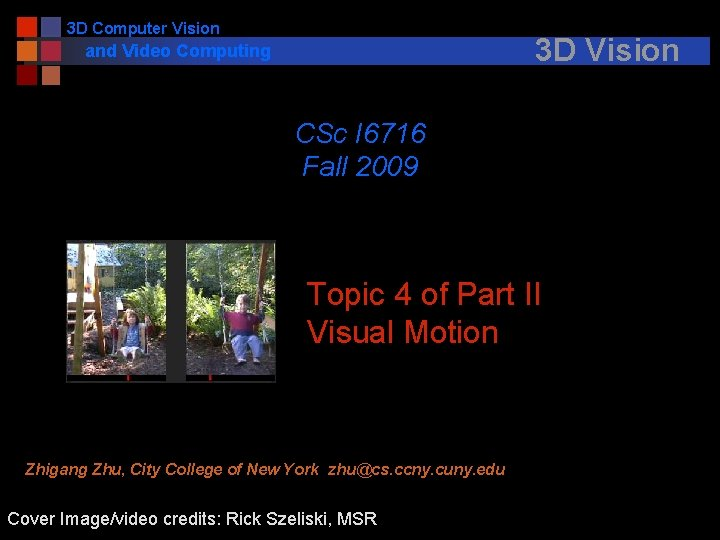3 D Computer Vision 3 D Vision and Video Computing CSc I 6716 Fall 2009 Topic 4 of Part II Visual Motion Zhigang Zhu, City College of New York [email protected] ccny. cuny. edu Cover Image/video credits: Rick Szeliski, MSR3 D Computer Vision and Video Computing n Problems and Applications l l n l l Optical flow equation and the aperture problem Estimating optical flow 3 D motion & structure from optical flow Feature-based Approach l l l n Basics – Notations and Equations Three Important Special Cases: Translation, Rotation and Moving Plane Motion Parallax Optical Flow l n The importance of visual motion Problem Statement The Motion Field of Rigid Motion l n Outline of Motion Two-frame algorithm Multi-frame algorithm Structure from motion – Factorization method Advanced Topics l l l Spatio-Temporal Image and Epipolar Plane Image Video Mosaicing and Panorama Generation Motion-based Segmentation and Layered Representation3 D Computer Vision and Video Computing The n Structure from Motion l Apparent motion is a strong visual clue for 3 D reconstruction n n More than a multi-camera stereo system Recognition by motion (only) l Biological visual systems use visual motion to infer properties of 3 D world with little a priori knowledge of it n n Importance of Visual Motion Blurred image sequence Visual Motion = Video ! [Go to CVPR 2004 -2009 Sites for Workshops] l Video Coding and Compression: MPEG 1, 2, 4, 7… l Video Mosaicing and Layered Representation for IBR l Surveillance (Human Tracking and Traffic Monitoring) l HCI using Human Gesture (video camera) l Image-based Rendering l …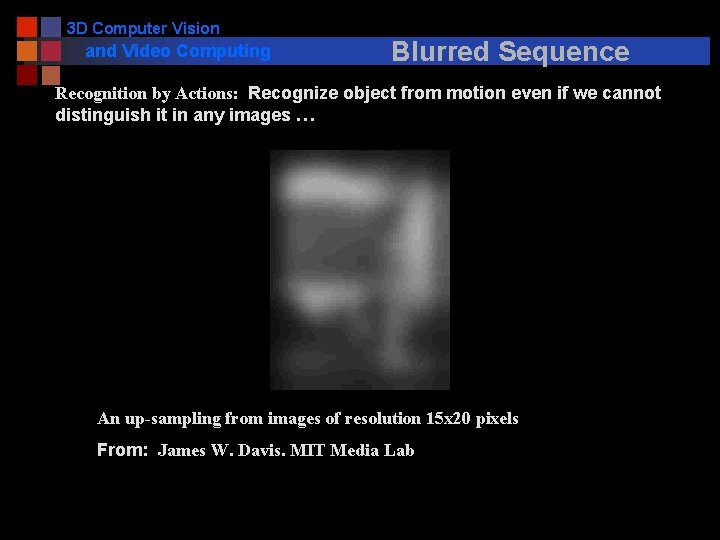3 D Computer Vision and Video Computing Blurred Sequence Recognition by Actions: Recognize object from motion even if we cannot distinguish it in any images … An up-sampling from images of resolution 15 x 20 pixels From: James W. Davis. MIT Media Lab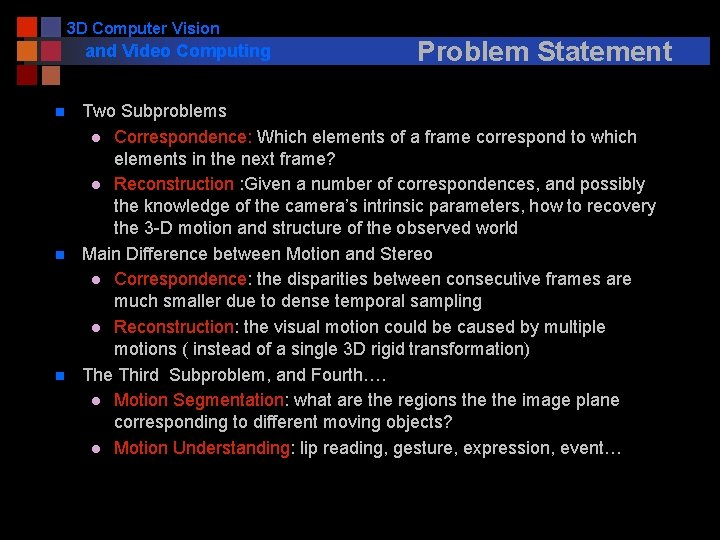3 D Computer Vision and Video Computing n n n Problem Statement Two Subproblems l Correspondence: Which elements of a frame correspond to which elements in the next frame? l Reconstruction : Given a number of correspondences, and possibly the knowledge of the camera’s intrinsic parameters, how to recovery the 3 -D motion and structure of the observed world Main Difference between Motion and Stereo l Correspondence: the disparities between consecutive frames are much smaller due to dense temporal sampling l Reconstruction: the visual motion could be caused by multiple motions ( instead of a single 3 D rigid transformation) The Third Subproblem, and Fourth…. l Motion Segmentation: what are the regions the image plane corresponding to different moving objects? l Motion Understanding: lip reading, gesture, expression, event…3 D Computer Vision and Video Computing n Two Subproblems l Correspondence: n n l Differential Methods - >dense measure (optical flow) Matching Methods -> sparse measure Reconstruction : More difficult than stereo since n n n Approaches Motion (3 D transformation betw. Frames) as well as structure needs to be recovered Small baseline causes large errors The Third Subproblem l Motion Segmentation: Chicken and Egg problem n Which should be solved first? Matching or Segmentation n n Segmentation for matching elements Matching for Segmentation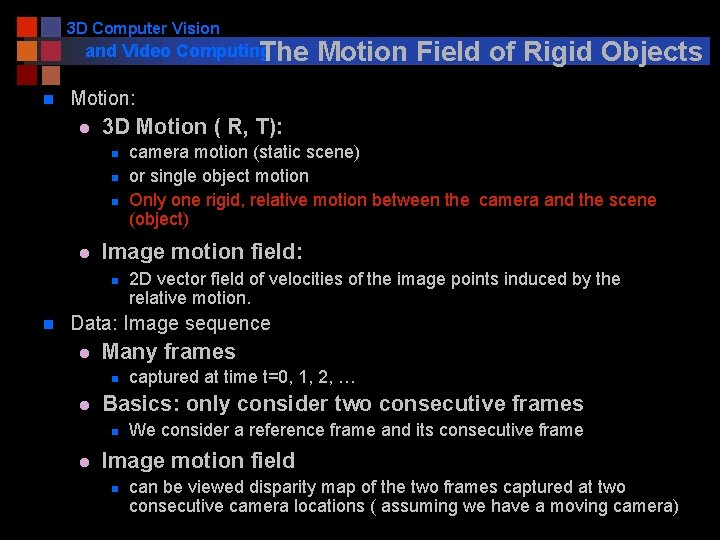3 D Computer Vision and Video Computing The n Motion: l 3 D Motion ( R, T): n n n l camera motion (static scene) or single object motion Only one rigid, relative motion between the camera and the scene (object) Image motion field: n n Motion Field of Rigid Objects 2 D vector field of velocities of the image points induced by the relative motion. Data: Image sequence l Many frames n l Basics: only consider two consecutive frames n l captured at time t=0, 1, 2, … We consider a reference frame and its consecutive frame Image motion field n can be viewed disparity map of the two frames captured at two consecutive camera locations ( assuming we have a moving camera)3 D Computer Vision and Video Computing The Motion Field of Rigid Objects n Notations l P = (X, Y, Z)T: 3 -D point in the camera reference frame l p = (x, y, f)T : the projection of the scene point in the pinhole camera n Relative motion between P and the camera l T= (Tx, Ty, Tz)T: translation component of the motion l w=(wx, wy, wz)T: the angular velocity n P Note: l How to connect this with stereo geometry (with R, T)? l Image velocity v= ? p v Y X f O Z V3 D Computer Vision and Video Computing The Motion Field of Rigid Objects n Notations l P = (X, Y, Z)T: 3 -D point in the camera reference frame l p = (x, y, f)T : the projection of the scene point in the pinhole camera n Relative motion between P and the camera l T= (Tx, Ty, Tz)T: translation component of the motion l w=(wx, wy, wz)T: the angular velocity n Note: l How to connect this with stereo geometry (with R, T)?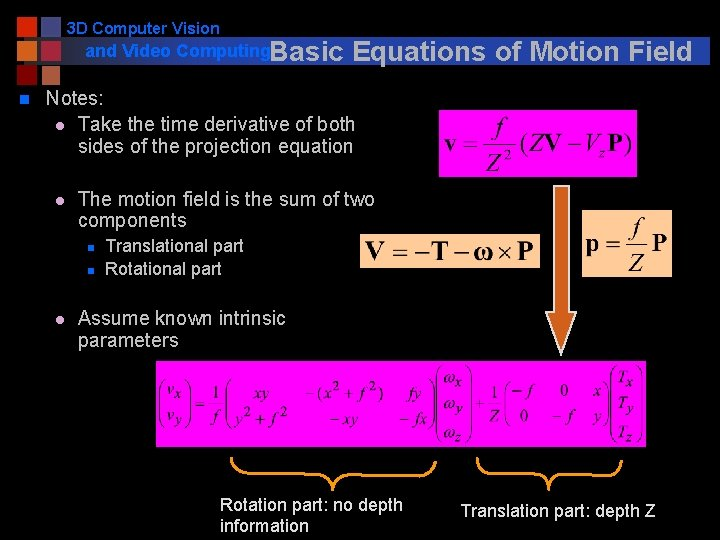3 D Computer Vision and Video Computing. Basic n Equations of Motion Field Notes: l Take the time derivative of both sides of the projection equation l The motion field is the sum of two components n n l Translational part Rotational part Assume known intrinsic parameters Rotation part: no depth information Translation part: depth Z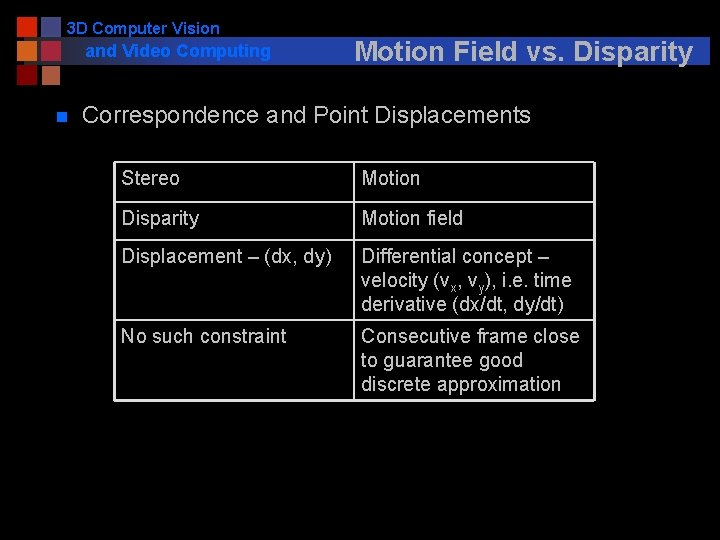3 D Computer Vision and Video Computing n Motion Field vs. Disparity Correspondence and Point Displacements Stereo Motion Disparity Motion field Displacement – (dx, dy) Differential concept – velocity (vx, vy), i. e. time derivative (dx/dt, dy/dt) No such constraint Consecutive frame close to guarantee good discrete approximation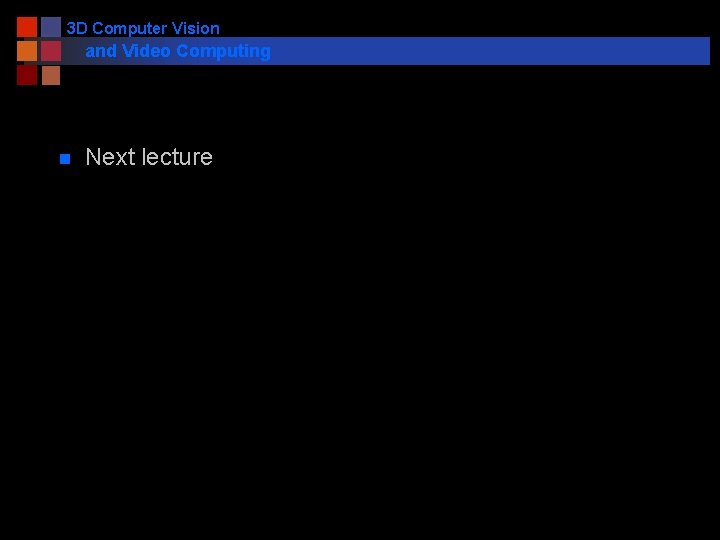3 D Computer Vision and Video Computing n Next lecture3 D Computer Vision and Video Computing Special n Pure Translation (w =0) n Radial Motion Field (Tz <> 0) l Vanishing point p 0 =(x 0, y 0)T : n l Vectors towards p 0 if Tz > 0 Depth estimation n n Vectors away from p 0 if Tz < 0 FOC (focus of contraction) n l motion direction FOE (focus of expansion) n l Case 1: Pure Translation depth inversely proportional to magnitude of motion vector v, and also proportional to distance from p to p 0 Parallel Motion Field (Tz= 0) l Depth estimation: n depth inversely proportional to magnitude of motion vector v Tz =03 D Computer Vision and Video Computing Special Case 2: Pure Rotation n Pure Rotation (T =0) l Does not carry 3 D information n Motion Field (approximation) l Small motion l A quadratic polynomial in image coordinates (x, y, f)T n Image Transformation between two frames (accurate) l Motion can be large l Homography (3 x 3 matrix) for all points n Image mosaicing from a rotating camera l 360 degree panorama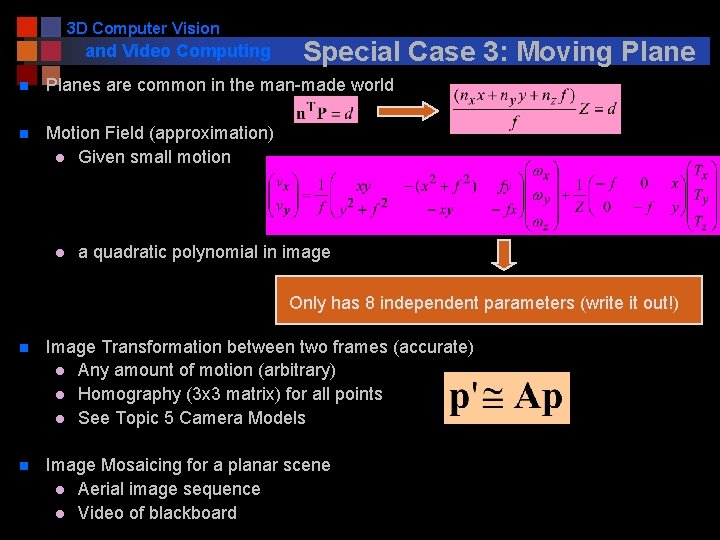3 D Computer Vision and Video Computing Special Case 3: Moving Plane n Planes are common in the man-made world n Motion Field (approximation) l Given small motion l a quadratic polynomial in image Only has 8 independent parameters (write it out!) n Image Transformation between two frames (accurate) l Any amount of motion (arbitrary) l Homography (3 x 3 matrix) for all points l See Topic 5 Camera Models n Image Mosaicing for a planar scene l Aerial image sequence l Video of blackboard3 D Computer Vision and Video Computing n Pure Translation l l n Vanishing point and FOE (focus of expansion) Only translation contributes to depth estimation Pure Rotation l l n Special Cases: A Summary Does not carry 3 D information Motion field: a quadratic polynomial in image, or Transform: Homography (3 x 3 matrix R) for all points Image mosaicing from a rotating camera Moving Plane l l l Motion field is a quadratic polynomial in image, or Transform: Homography (3 x 3 matrix A) for all points Image mosaicing for a planar scene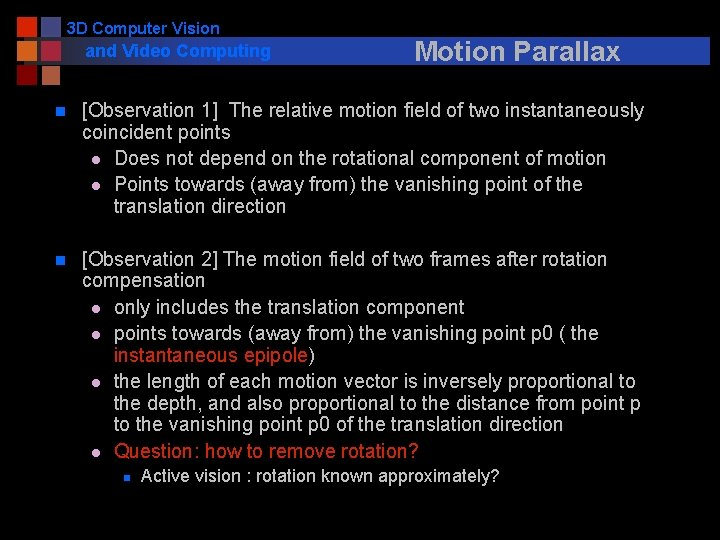3 D Computer Vision and Video Computing Motion Parallax n [Observation 1] The relative motion field of two instantaneously coincident points l Does not depend on the rotational component of motion l Points towards (away from) the vanishing point of the translation direction n [Observation 2] The motion field of two frames after rotation compensation l only includes the translation component l points towards (away from) the vanishing point p 0 ( the instantaneous epipole) l the length of each motion vector is inversely proportional to the depth, and also proportional to the distance from point p to the vanishing point p 0 of the translation direction l Question: how to remove rotation? n Active vision : rotation known approximately?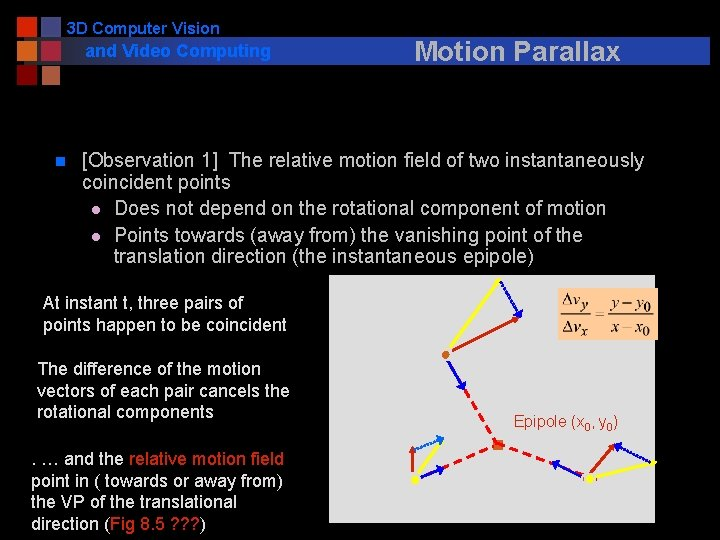3 D Computer Vision and Video Computing n Motion Parallax [Observation 1] The relative motion field of two instantaneously coincident points l Does not depend on the rotational component of motion l Points towards (away from) the vanishing point of the translation direction (the instantaneous epipole) At instant t, three pairs of points happen to be coincident The difference of the motion vectors of each pair cancels the rotational components. … and the relative motion field point in ( towards or away from) the VP of the translational direction (Fig 8. 5 ? ? ? ) Epipole (x 0, y 0)3 D Computer Vision and Video Computing n Motion Parallax [Observation 2] The motion field of two frames after rotation compensation l only includes the translation component l points towards (away from) the vanishing point p 0 ( the instantaneous epipole) l the length of each motion vector is inversely proportional to the depth, l and also proportional to the distance from point p to the vanishing point p 0 of the translation direction (if Tz <> 0) Question: how to remove rotation? n n Active vision : rotation known approximately? Rotation compensation can be done by image warping after finding three (3) pairs of coincident points p 0 v p FOE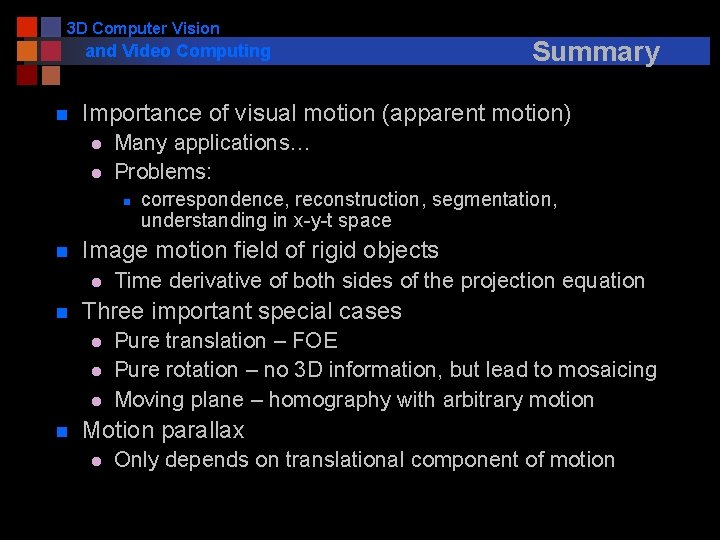3 D Computer Vision and Video Computing n Importance of visual motion (apparent motion) l l Many applications… Problems: n n Time derivative of both sides of the projection equation Three important special cases l l l n correspondence, reconstruction, segmentation, understanding in x-y-t space Image motion field of rigid objects l n Summary Pure translation – FOE Pure rotation – no 3 D information, but lead to mosaicing Moving plane – homography with arbitrary motion Motion parallax l Only depends on translational component of motion3 D Computer Vision and Video Computing n Next lecture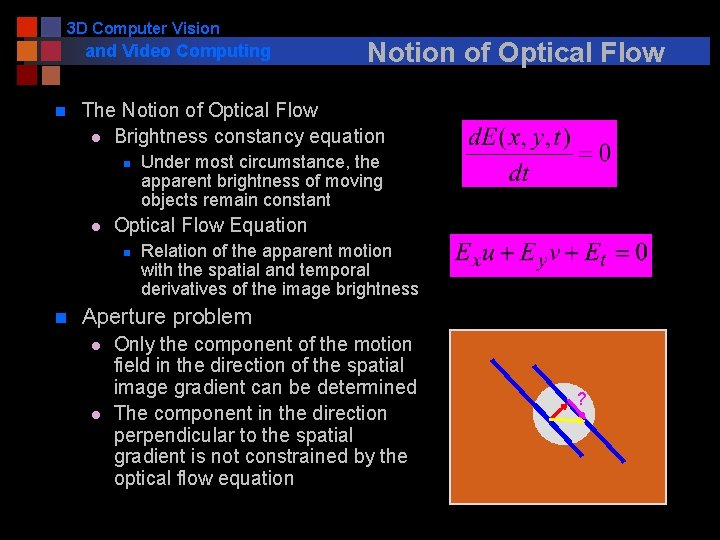3 D Computer Vision and Video Computing n The Notion of Optical Flow l Brightness constancy equation n l Under most circumstance, the apparent brightness of moving objects remain constant Optical Flow Equation n n Notion of Optical Flow Relation of the apparent motion with the spatial and temporal derivatives of the image brightness Aperture problem l l Only the component of the motion field in the direction of the spatial image gradient can be determined The component in the direction perpendicular to the spatial gradient is not constrained by the optical flow equation ?3 D Computer Vision and Video Computing n Constant Flow Method l l l n Estimating Optical Flow Assumption: the motion field is well approximated by a constant vector within any small region of the image plane Solution: Least square of two variables (u, v) from Nx. N Equations – Nx. N (=5 x 5) planar patch Condition: ATA is NOT singular (null or parallel gradients) Weighted Least Square Method l l Assumption: the motion field is approximated by a constant vector within any small region, and the error made by the approximation increases with the distance from the center where optical flow is to be computed Solution: Weighted least square of two variables (u, v) from Nx. N Equations – Nx. N patch n Affine Flow Method l l Assumption: the motion field is well approximated by a affine parametric model u. T = Ap. T+b (a plane patch with arbitrary orientation) Solution: Least square of 6 variables (A, b) from Nx. N Equations – Nx. N planar patch3 D Computer Vision and Video Computing n Using Optical Flow 3 D motion and structure from optical flow (p 208 - 212) l Input: n n l Intrinsic camera parameters dense motion field (optical flow) of single rigid motion Algorithm n ( good comprise between ease of implementation and quality of results) n Stage 1: Translation direction n n Epipole (x 0, y 0) through approximate motion parallax Key: Instantaneously coincident image points Approximation: estimating differences for ALMOST coincident image points Stage 2: Rotation flow and Depth n n Knowns: flow vector, and direction of translational component One point, one equation (without depth)– w n l Least square approximation of the rotational component of flow From motion field to depth Output n n n Direction of translation (f Tx/Tz, f Ty/Tz, f) = (x 0, y 0, f) Angular velocity 3 -D coordinates of scene points (up to a common unknown scale)3 D Computer Vision and Video Computing n n n Some Details Step 1. Get (Tx, Ty, Tz) = s (x 0, y 0, f) Step 2. For every point (x, y, f) with known v, get one equation about w from the motion equation (by eliminate Z since it’s different from point to point) Step 3. Get Z (up to a scale s) given T/s and w Rotation part: no depth information Translation part: depth Z3 D Computer Vision and Video Computing n Two frame method - Feature matching l An Algorithm Based on the Constant Flow Method n n n Features – corners detection by observing the coefficient matrix of the spatial gradient evaluation (2 x 2 matrix ATA) Iteration approach: estimation – warping – comparison Multiple frame method - Feature tracking l Kalman Filter Algorithm n n n Feature-Based Approach Estimating the position and uncertainty of a moving feature in the next frame Two parts: prediction (from previous trajectory) and measurement from feature matching Using a sparse motion field l 3 D motion and structure by feature tracking over frames l Factorization method n n n Orthographic projection model Feature tracking over multiple frames SVD3 D Computer Vision and Video Computing Motion-Based Segmentation n Change Detection l Stationary camera(s), multiple moving subjects l Background modeling and updating l Background subtraction l Occlusion handling n Layered representation (I)– rotating camera l Rotating camera + Independent moving objects l Sprite - background mosaicing l Synopsis – foreground object sequences n Layered representation (II)– translating (and rotating) camera l Arbitrary camera motion l Scene segmentation into layers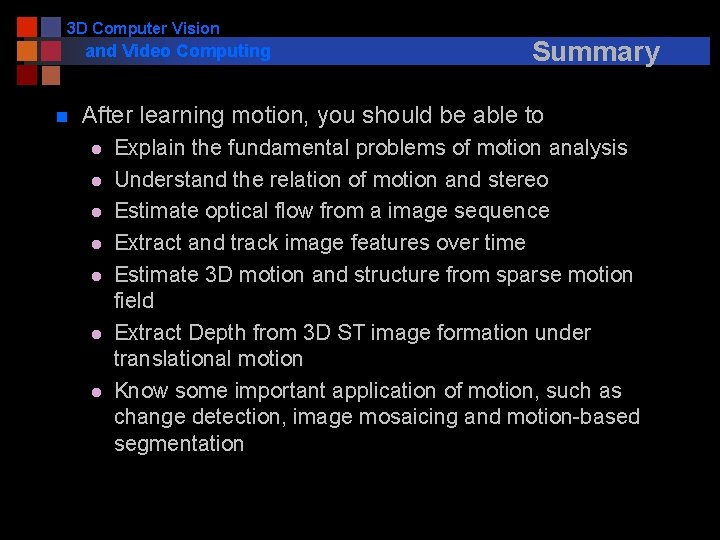3 D Computer Vision and Video Computing n Summary After learning motion, you should be able to l l l l Explain the fundamental problems of motion analysis Understand the relation of motion and stereo Estimate optical flow from a image sequence Extract and track image features over time Estimate 3 D motion and structure from sparse motion field Extract Depth from 3 D ST image formation under translational motion Know some important application of motion, such as change detection, image mosaicing and motion-based segmentation3 D Computer Vision and Video Computing n Reviews, Exam and Projects Exam & Project Presentations n. Homework #4 due in two weeks Next